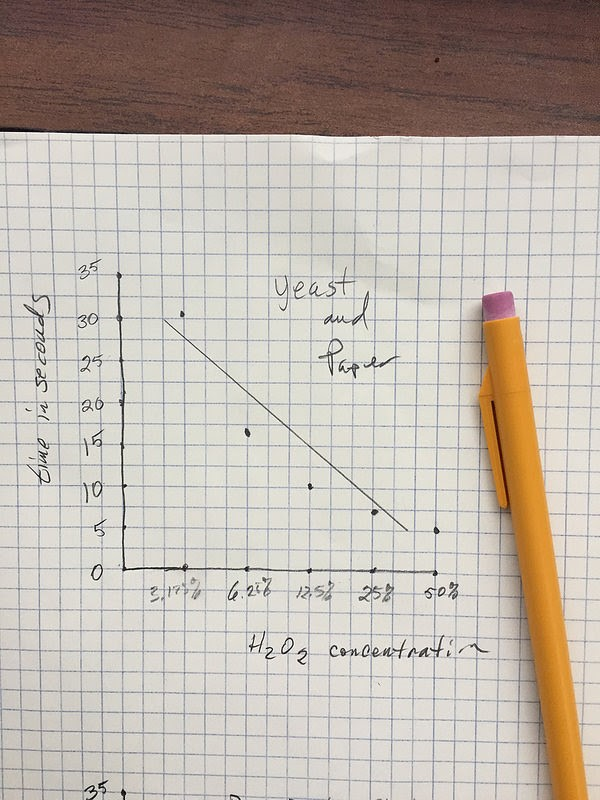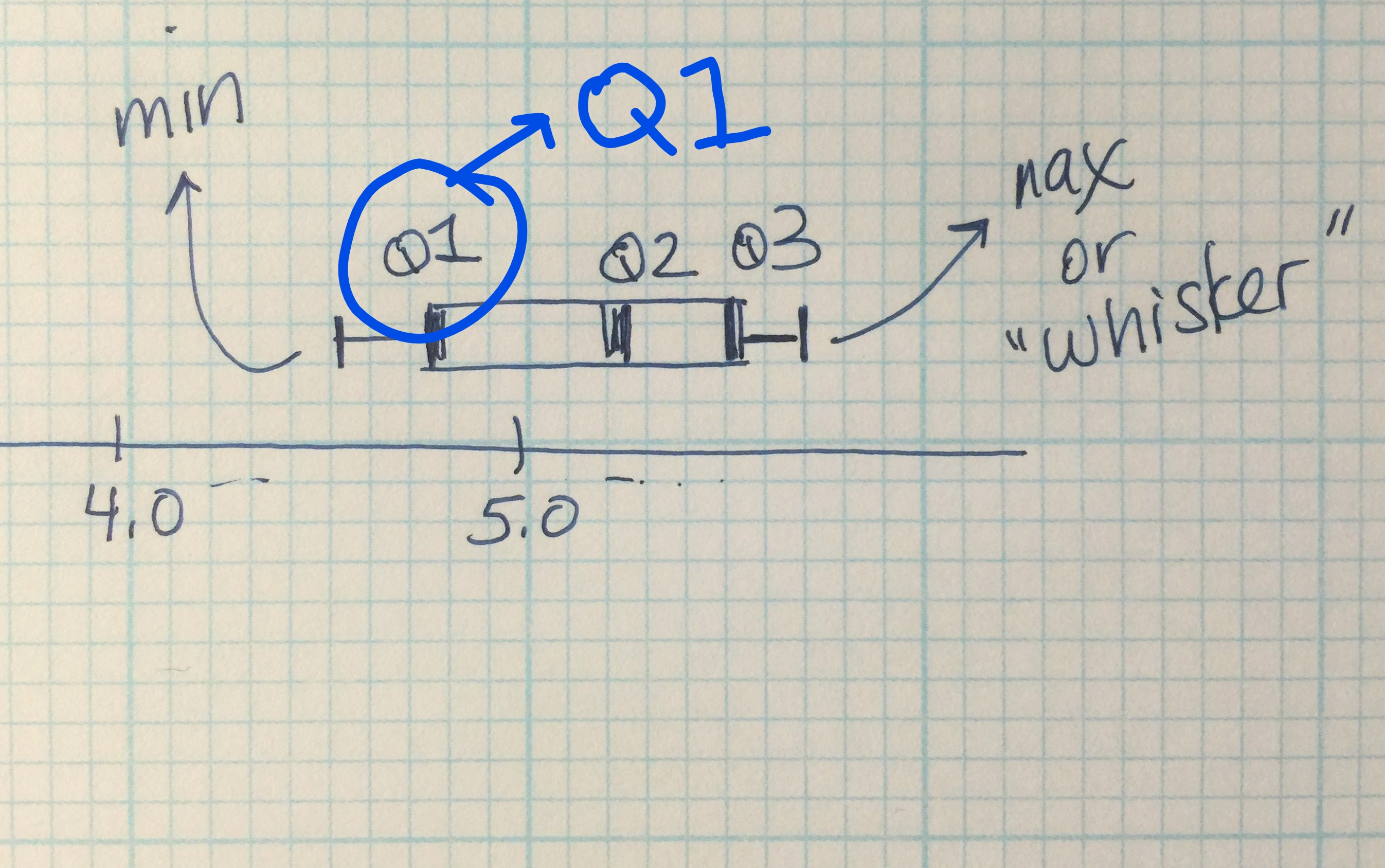# Lab#5: Enzymes/Data Analysis

Analyzing the data from Yeast Catalase Lab

1. Introduction to data analysis

Here’s some sample data from another teacher:First of all, is this table of data properly labeled? Does it make sense? Do we really want the separate results for each disk? Why would you want to see the individual data points first before crunching and summarizing? Just as a small hint, look at the numbers for disk#3.

Thoughts?

The statement that it is “way off” implies a quantitative relationship. How do you decide that? What do you do about that point? Do you keep it? Do you ignore it? Throw it away? If you think you can justify removing this piece of data, do so, but ethically, you would have to report that you did and provide the rationale (perhaps in an appendix).

Can you re-label the data into a new table? (don’t look at the next table until you do)Short discussion of what the “mean” means: The fact is the parameter we are trying to estimate or measure is the mean of the population distribution. In other words there is a distribution that we are trying to determine and we will always be measuring that distribution of possibilities. This idea was one of the big outcomes of the development of statistics in the early 1900’s and can be credited to Karl Pearson. Today, in science, the measurement and such assume these distributions–even when measuring some physical constant like the acceleration of gravity. That wasn’t the case in the 1800’s and many folks today think that we are measuring some precise point when we collect our data.

2. Graphing
How would you graph your data? How would you space the hydrogen peroxide concentrations? Just do a simple paper graph on grid paper (take a picture for your lab notebooks or paste it directly in).

Here’s a quick paper graph straight from the table.Note also, that this hypothetical student added a “best fit” line. Nice fit but does it fit the trend in the actual data? Is there actually a curve? This is where referring back to the models covered earlier can really pay off. What kind of curve would you expect? When we drop a disk in the H2O2 and time how long it rises are we measuring how long the reaction takes place or are we measuring a small part of the overall reaction? At this point it would be good to consider what is going on. The reaction is continuing long after the disk has risen as evidenced by all the bubbles that have accumulated in this image. So what is the time of disk rise measuring? Let’s return to that in a bit but for now let’s look at some more student work.

What about the independent variable? Which axis should that be? And the dependent variable, or perhaps a better name, the explanatory variable?

What “message” are we trying to convey with our graph? What is the simplest graphic that can convey the message? What can enhance that message? What is my target audience?

How can you show the variability of the data in a graph? You could plot ALL the data points (instead of using the mean), but is there a simpler way to do that?

1. Box plots: The nice thing about box plots is that they capture the range and variability in the data. With a plot like this you can plainly see that there is really little or no overlap of data between the treatments and you can also see a trend.

If you are new to box plots, I highly suggest the link to the right at Purple Math; the link will walk you through the details of making a box plot. It is good practice to make them by hand at first (instead of relying on a spreadsheet) so you really understand how the data is used to show the variability and mean/medians. I’ll work through the sample data to provide an example.

The first group of data points are the 1.5% H2O2 results: 4.65, 5.65, 4.78, 5.18, 5.27, 5.07, 5.45, 5.35
You need to re-order them from least to greatest: 4.65, 4.78, 5.07, 5.18, 5.27, 5.35, 5.45, 5.65,

Now find the median for this group, which divides the list into two halves: 4.65, 4.78, 5.07, 5.18,      5.27, 5.35, 5.45, 5.65,
Because we have an even number of numbers, the median will be the mean of the 2 middle numbers: 5.18 and 5.27 = 5.23
This is also called Q2.

To divide the data into quarters, you then find the medians of these two halves. Now, we have three numbers (odd), so we just take the middle number, ignoring the numbers that were previously used to calculate the median, or Q2.
Q1 = median of the first half of the numbers = 4.78
Q3 = median of the second half of the numbers = 5.45

We also need the min and max, which are 4.65 and 5.65, respectively.
Now we can graph!

The “box” part of the plot goes from Q1 to Q3:Note that the “whiskers” are drawn to the endpoints.

Here is all the crunched data for the sample data:And here is a hand drawn graph with all of the data:Some helpful links on making box plots and scatterplots:

http://www.purplemath.com/modules/boxwhisk.htm

What is bivariate data?

Bivariate data can be categorical-categorical, categorical-quantitative, or quantitative-quantitative. Here is an example of each combination, along with the visualization method that Landy mentioned, and the appropriate hypothesis test.

– *C-C*: ​gender explains (is associated with) political party. We visualize this with segmented or side-by-side bar graphs. We test this with Chi-square tests for two-way tables.
– *C-Q*: gender explains (is associated with) height. We visualize this with parallel dotplots, among other graphs. We test this with a 2-sample t-test. (If the categorical variable has more than 2 categories, we test this with a One-way ANOVA F test, which is not in the AP curriculum.)
– *Q-Q*: foot length explains (is associated with) height. We visualize this with scatterplots. We test this with a t-test for slope.

This is just a quick summary of beginning stats, and you won’t need to know all this for the AP Biology exam. But if you are interested in more detail, find a good statistics book, or start with some beginning statistics videos here:
Intro to Box Plots: https://www.youtube.com/watch?v=KzVvo0u__-o
Intro to regression and scatterplots: https://www.youtube.com/watch?feature=player_detailpage&v=TGxseSNW_-0

All of “APStatsGuys” videos: https://www.youtube.com/watch?feature=player_detailpage&v=TGxseSNW_-0
He’s an AP Stats teacher who posts good learning videos on You Tube.

If you are interested in a good stats software that many AP Statistics teachers use, try Fathom: https://fathom.concord.org/download/
There are a lot of resources available for free online, just search for Fathom AP Stats. If you want something that statisticians use in their work every day, there is also the programming language called R (free download: http://www.r-project.org/). As a language, there’s a steep learning curve, but with some practice, you can quickly do any kind of statistical analysis you’d like. Here are some free learning videos from another stats teacher:
His playlist for AP Stats: https://www.youtube.com/playlist?list=PLzpuTaStf5rRF_2Cu72kMgCh2CD-c7Tdw

2. Scatterplot: a way to plot every point and the mean.

Why is this method superior to box plots? It isn’t superior to box plots for every case, it is another tool in your arsenal to try and make sense of the data and present your interpretation of what is happening in the experiment. Statisticians say that scatterplots are best used when you have two continuous variables (they both are a range of numbers, or quantitative data), so you can find correlation and regression if needed. A box plot is best when you have a continuous variable and a categorical variable (like colors of leaves, or kind of animal). These are both ways of dealing with bivariate data.

How do you do it?

You plot every point on the graph, and to give a sense of the scatter, you also plot the mean of each set of data as a larger dot. This is actually pretty similar to a box plot in that you have something representing the mean (the box or the main dot) and then the whiskers (minimum and maximum) and the actual data.

I’m not going to go into detail about how to arrange your data in order to use a statistical software package or Excel or a graphing calculator; I’m going to go through it and do it by hand. Always a good idea to make graphs by hand for a while before switching to Excel/graphing program so you know exactly what is involved.

This is a properly scaled scatterplot (bivariate plot) that demonstrates how the response (or dependent) variable (time to rise) varies according to the explanatory (or independent) variable (H2O2  concentration).  It might seem like the H2O2 concentrations isn’t continuous (or quantitative) because we chose those particular dilutions, but they are still numbers on a scale.Quick question: “What’s up with time being the dependent variable?”  Why is it on the y-axis?